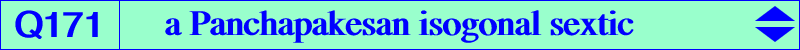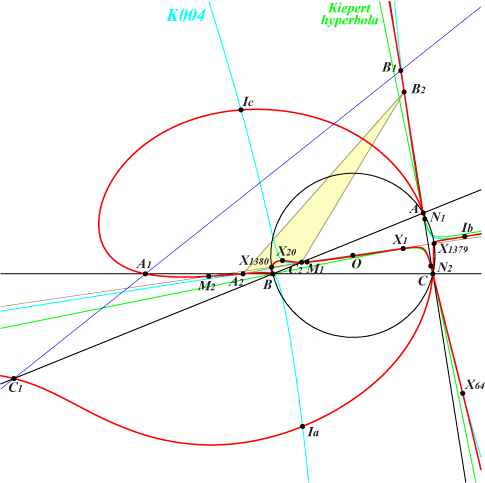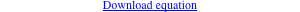too complicated to be written here. Click on the link to download a text file.X(1), X(20), X(64), X(1379), X(1380), X(3413), X(3414), X(39641), X(39642) X(39641), X(39642) are the imaginary foci of the Brocard inellipse, on the Brocard axis and the Kiepert hyperbola, see Table 79 excenters A1, B1, C1 : traces of the trilinear polar of X(3) A2, B2, C2 : cevians of X(20) M1, M2 mentioned at Q170 and their isogonal conjugates N1, N2 anti-points, see Table 77See Q170 for explanations. A, B, C are double points with tangents passing through X(64) and the corresponding traces of the orthic axis on the sidelines of ABC. Q171 meets the Kiepert hyperbola at 12 points : A, B, C (each twice), X(3413), X(3414), X(39641), X(39642), N1, N2. Q171 meets the Darboux cubic K004 at 18 points : A, B, C (each thrice), in/excenters, X(20), X(64), A2, B2, C2. Q171 meets the McCay cubic K003 at 18 points : A, B, C (each twice), in/excenters, X(39641), X(39642), anti-points.